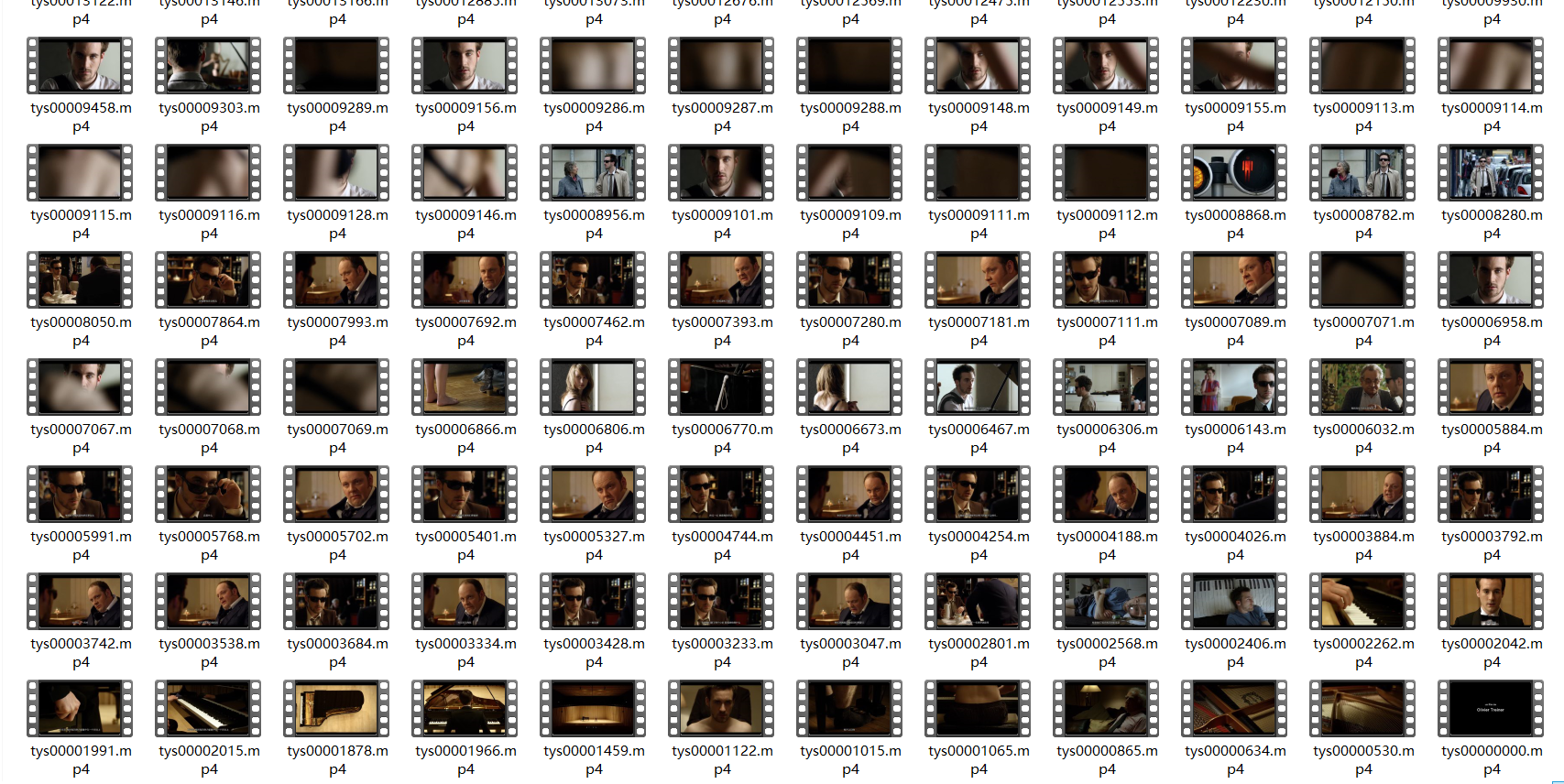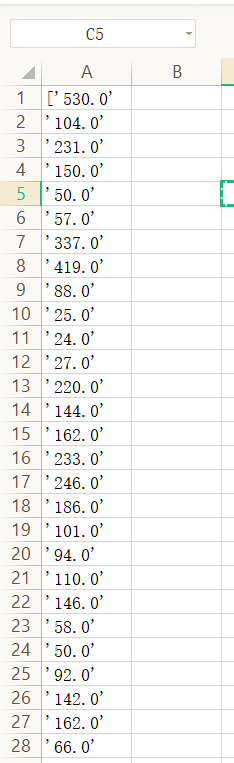# 半自动拉片机

## 所需工具

PythonFFmpeg

（Python中需安装CV2和ffmpeg库，推荐使用pip安装）

## 代码部分

``````import os,time
import cv2
import ffmpy

#获取文件夹中的文件名列表
def get_file_list(file_path):
dir_list = os.listdir(file_path)
if not dir_list:
return
else:
# 注意，这里使用lambda表达式，将文件按照最后修改时间顺序升序排列
# os.path.getmtime() 函数是获取文件最后修改时间
# os.path.getctime() 函数是获取文件最后创建时间
dir_list = sorted(dir_list,key=lambda x: os.path.getmtime(os.path.join(file_path, x)))
# print(dir_list)
return dir_list
# 每个镜头的地址
file_path = 'E:/DaVinciobject/114514/'

dir_list = get_file_list(file_path)
``````
``````#读取时长
def get_vedio_height_width(filename):
cap = cv2.VideoCapture(filename)
time = str(cap.get(cv2.CAP_PROP_FRAME_COUNT))
return time

# print(listdir)

``````
``````list=[]
def main():
for dir in dir_list:
time = get_vedio_height_width(file_path+dir)
a=time
line=a
list.append(line)
b=str(list)
print(b)
# 将数据写入临时文件
with open("2233.txt","w") as f:
f.write(b)
s=[]
# 将数据分行
f  = open('H:\\test\\2233\\2233.txt','r', encoding='utf-8')
for lines in f:
ls = lines.strip('\n').replace(' ','').replace('《','/').replace('?','').split(',')
for i in ls:
s.append(i)
f.close()
# 将数据写入csv文件
f1 = open('2233.csv','w',encoding='utf-8')
for j in s:
f1.write(j+'\n')
f1.close()
# 删除临时文件
os.remove("./2233.txt")
Shu_Zu_Shu=len(list)
# print(Shu_Zu_Shu)
i=1
print(Shu_Zu_Shu)
#截取第1帧
for dir in dir_list:
ff = ffmpy.FFmpeg(
inputs={file_path+dir: None},
# outputs={mingming+'.mp4': None}
outputs={file_path+dir+'.jpg': '-ss 0 -frames:v 1'}
)
ff.run()
main()
``````

## 效果展示（运行过程不展示了，但必须要说，跑循环截图的时候控制台一串串信息往下跑是真的帅）`'`可以在文件中按`ctrl+f`批量去掉）